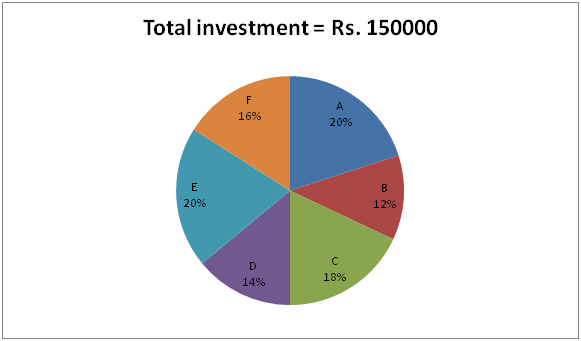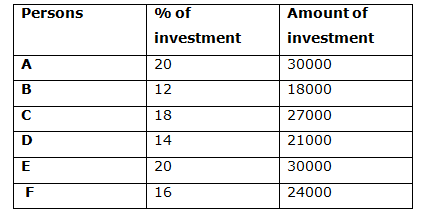# IBPS PO Mains 2018 – Quantitative Aptitude Questions Day-13

Dear Readers, Bank Exam Race for the Year 2018 is already started, To enrich your preparation here we have providing new series of Practice Questions on Quantitative Aptitude – Section. Candidates those who are preparing for IBPS PO Mains 2018 Exams can practice these questions daily and make your preparation effective.

[WpProQuiz 4109]

1) 3 years ago, the ratio of age of A and B is 2: 3. After 8 years, the sum of the ages of A and B is 97 years. C is 5 years elder to D. The average of present ages of A, B, C and D is 45 years. Find the present ages of C and D?

a) 48 years and 36 years

b) 50 years and 42 years

c) 52 years and 47 years

d) 45 years and 35 years

e) None of these

2) If the difference between the discount of 35% and two successive discounts of 28% and 20% is equal to Rs. 888, then find the cost price of article if the shopkeeper marked up the price by 25%?

a) Rs. 10200

b) Rs. 9600

c) Rs. 11400

d) Rs. 8500

e) None of these

3) A boat takes 27 hours for travelling downstream from point A to point B and coming back (upstream) to point C midway between A and B. If the velocity of the stream is 12 km/hr and the speed of the boat in still water is 28 km/hr, then find the distance between A to B?

a) 360 km

b) 520 km

c) 480 km

d) 440 km

e) None of these
4) A Circular path is surrounding the circular plot is being gravelled at a total cost of Rs. 5390 at Rs. 5 per square meter. Find the width of the path, if the radius of the circle plot is 21 m?

a) 8 m

b) 10 m

c) 9 m

d) 7 m

e) None of these

5) In an examination, Manik scored 38% marks and failed by 16 marks. In the same examination, Rajesh scored 44% marks and get 14 marks more than the passing marks. What is the score of Mohan in the same examination, who secured 65 % total marks?

a) 350

b) 380

c) 325

d) 295

e) None of these

Directions (Q. 6 – 10) Study the following information carefully and answer the given questions.

The following pie chart shows the percentage distribution of total investment of six different persons.6) A and C started a business. After 5 months, A invested Rs. 4000 more and at the same time G enter into a business by investing Rs. 5000 more than the investment of C. Find the share of G, if the total share of A and C together is Rs. 103240?

a) Rs. 35620

b) Rs. 48950

c) Rs. 32480

d) Rs. 41230

e) None of these

7) B and D together started a business. After 6 months, B left the business and at the same time E enters into a business. D left the business after 2 months of B left the business. E invested the amount for x months only. Out of the total profit of Rs. 72000 for the certain period, D received the share of Rs. 21000. Find the value of x?

a) 10

b) 9

c) 12

d) 8

e) None of these

8) B, F and N together started a business by investing in the ratio of 3: 4 : 6. After 7 months, N is replaced by D. And after another 2 months, B invested Rs. 6000 more. Find the total profit at the end of the year, if the share of B and D together is Rs. 113000?

a) Rs. 278000

b) Rs. 252000

c) Rs. 316000

d) Rs. 293000

e) None of these

9) B, E and F started a business. B is a working partner but E and F are sleeping partner in the business. For managing the business, B received 10% of the total profit. Find the difference between the share of B and F, if the total profit at the end of the year is Rs. 78000?

a) Rs. 2350

b) Rs. 1950

c) Rs. 2500

d) Rs. 1600

e) None of these

10) C, D and E together started a business. After 4 months, C withdraw one-third of the initial investment and after another 2 months, E withdraw one-sixth of the initial investment. Find the share of E, if the total profit at the end of the year is Rs. 139000?

a) Rs. 63000

b) Rs. 48000

c) Rs. 55000

d) Rs. 52000

e) None of these

3 years ago, the ratio of age of A and B = 2: 3 (2x, 3x)

After 8 years, the sum of the ages of A and B = 97

= > 2x + 11 + 3x + 11 = 97

= > 5x + 22 = 97

= > 5x = 75

= > x = 15

The present age of A and B = 2x + 3, 3x + 3 = 33, 48

C = D + 5

C – D = 5 —à (1)

The average of present ages of A, B, C and D = 45 years

The total present ages of A, B, C and D = 45*4 = 180

= > 33 + 48 + C + D = 180

= > C + D = 180 – 81

= > C + D = 99 –à(2)

By solving of equation (1) and (2), we get

C = 52, D = 47

The present age of C and D = 52 years and 47 years

Let the marked price be x,

According to the question,

x*(65/100) – X*(72/100)*(80/100) = 888

(13x/20) – (72x/125) = 888

(1625x – 1440x)/(125*20) = 888

185x/2500 = 888

X = 888*(2500/185) = Rs. 12000

(125/100)*CP = 12000

CP = 12000*(100/125) = Rs. 9600

Speed of downstream = 28 + 12 = 40 km/hr
Speed of upstream = 28 – 12 = 16 km/hr
Total time = 27 hours

If distance between A to B is x, then distance between B to C = x/2,
Time = Distance/Speed

x/40 + (x/2)/16 = 27

x/40 + x/32 = 27
72x/(40*32) = 27

x = (27*40*32)/72 = 480 Km

The distance between A to B = 480 km

Radius of the circular plot = 21 m

Area of the circular path = 5390/5 = 1078

Area of the path = π(r + x)2 – πr2 (Here x is the width of the path)

= > (22/7) [(21+x)2 – 212]

= > (22/7) [441 + 42x + x2 – 441]

= > (22/7) [42x + x2]

(22/7) [42x + x2] = 1078

42x + x2 = 1078*(7/22)

42x + x2 = 343

X2 + 42x – 343= 0

(x+49) (x – 7) = 0

X = 7 meter

The width of the path = 7 meter

Here passing marks equal. So,

= > 38 % of total marks + 16 = 44 % of total marks – 14

= > 16 + 14 = (44 – 38) % of total marks

= > 30 = 6 % of total marks

= > Total marks = 30*(100/6) = 500

Mohan’s mark = (65/100)*500 = 325

Directions (6-10):The investment of G = 27000 + 5000 = Rs. 32000

The share of A, C and G,

= > [30000*5 + 34000*7]: [27000*12]: [32000*7]

= > [150000 + 238000]: 324000: 224000

= > 388000: 324000: 224000

= > 97: 81: 56

The total share of A and C together = Rs. 103240

178’s = 103240

1’s = 580

The share of G = 56’s = Rs. 32480

The share of D = Rs. 21000

The share of B and E = 72000 – 21000 = Rs. 51000

The share of B, D and E,

= > [1500*12*6] : [1500*14*8] : [1500*20*x]

= > 18 : 28 : 5x

According to the question,

= > 28/(18 + 5x) = 21000/51000

= > 5x = 50

= > x = 10

B, F and N together started a business by investing in the ratio of = 3: 4: 6

The investment of N = (24000/4)*6 = Rs. 36000

The share of B, F, N and D is,

= > [18000*9 + 24000*3] : [24000*12] : [36000*7] : [21000*5]

= > 234000: 288000: 252000: 105000

= > 78: 96: 84: 35

The share of B and D together = Rs. 113000

113’s = 113000

1’s = 1000

Total profit = 293’s = Rs. 293000

The share of B, E and F

= > 18000: 30000: 24000

= > 3: 5: 4

B = 78000*(10/100) = Rs. 7800

The share of B = 7800 + (70200/12)*3 = 7800 + 17550 = Rs. 25350

The share of E = (70200/12)*5 = Rs. 29250

The share of F = (70200/12)*4 = Rs. 23400

Required difference = 25350 – 23400 = Rs. 1950

The share of C, D and E

= > [27000*4 + 27000*(2/3)*8]: [21000*12]: [30000*6 + 30000*(5/6)*6]

= > [108000 + 144000]: : [180000 + 150000]

= > 252000: 252000: 330000

= > 84: 84: 110 = 42: 42: 55

139’s = 139000

1’s = 1000

The share of E = 55’s = Rs. 55000

Daily Practice Test Schedule | Good Luck

 Topic Daily Publishing Time Daily News Papers & Editorials 8.00 AM Current Affairs Quiz 9.00 AM Current Affairs Quiz (Hindi) 9.30 AM IBPS Clerk Prelims – Reasoning 10.00 AM IBPS Clerk Prelims – Reasoning (Hindi) 10.30 AM IBPS Clerk Prelims – Quantitative Aptitude 11.00 AM IBPS Clerk Prelims – Quantitative Aptitude (Hindi) 11.30 AM Vocabulary (Based on The Hindu) 12.00 PM IBPS PO Prelims – English Language 1.00 PM SSC Practice Questions (Reasoning/Quantitative aptitude) 2.00 PM IBPS PO/Clerk – GK Questions 3.00 PM SSC Practice Questions (English/General Knowledge) 4.00 PM Daily Current Affairs Updates 5.00 PM IBPS PO Mains – Reasoning 6.00 PM IBPS PO Mains – Quantitative Aptitude 7.00 PM IBPS PO Mains – English Language 8.00 PM